### AP Physics 1 Week 11 (Aug 20 - Aug 26, 2017)

- The last week before school starts, and the really weird problems-

Part 1:  Algebra (Rearrange these equations for Black Holes)

1.  Solve for c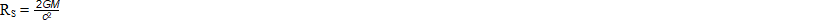2.  Solve for RS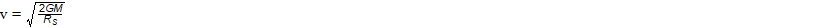3.  h-bar is a special constant, treat it as one variable, solve for c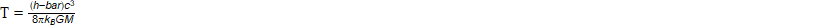4.  h-bar is a special constant, treat it as one variable, solve for M5. h-bar is a special constant, treat as one variable, solve for G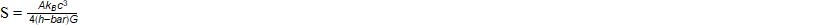Part 2:  Algebra (A reminder of the imaginary number i))
​The imaginary number i, is the square root of negative one.

Solve:

6.  i2

7.  i3

8.  i4

9.  i5

10.  ii (hint:  use your TI-8x calculator)

Part 3: Geometry/Trigonometry
Find the surface area and volume for each multi-dimensional structure below.  The radius, if circular is the value i, as well as the length, width, and height are also equal to i. Leave all answers in terms of i and
p.

11.  rectangular prism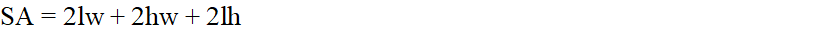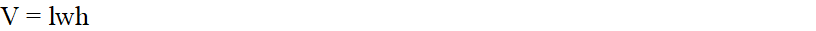12.  right circular cylinder (can)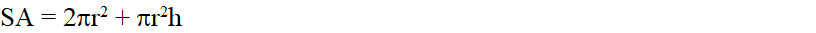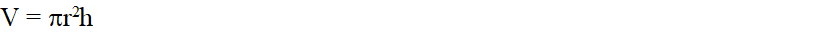13.  square pyramid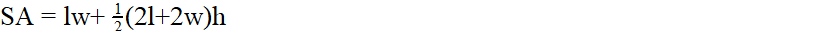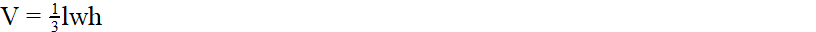14.  right circular cone (ice cream cone)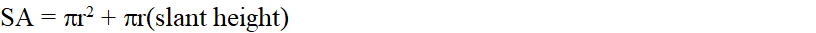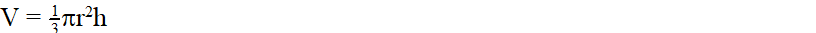15.  sphere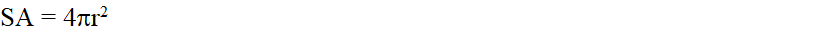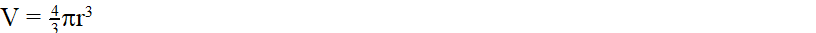Part 4:  Word Problems

Time Problems:

For questions 1 - 3  use a basic clock with two hands:
16.  How many minutes after 5 o'clock will the hands of a clock be together?
(WKP, p121 # 18)

17.  How soon after 7 o'clock will the hour and minute hand be together?
(WKP, p121 # 19)

18.  How soon after 8 o'clock will the hands of a clock first be opposite each other?
(WKP, p121 # 20)

Timelords from the planet Gallifrey developed clocks based on the base 10 metric scale rather than our earthly base 12 scale from the Babylonian system.  A day on the Earth is equal in duration to one day on Gallifrey.  On the Earth, 1 earth day is 24 earth hours, 1 earth hour is 60 earth minutes, and 1 earth minute is 60 earth seconds.  On Gallifrey, 1 gallifrean day is 10 gallifrean hours, 1 gallifrean hour is 100 gallifrean minutes, 1 gallifrean minute is 1000 gallifrean seconds.  Answer the following questions 4 -14:

19.  Which is larger, 1 earth hour or one gallifrean hour?

20.  Which is larger, 1 earth minute or one gallifrean minute?

21.  Which is larger, 1 earth second or one gallifrean second?

22 - 29 (a - h).  What  would the gallifrean clock read if the earth clock reads:
a.  3 a.m.
b.  6 a.m.
c.  9 a.m.
d. 12 p.m.
e.  3 p.m.
f.   6 p.m.
g.  9 p.m.
h. 12 a.m.

The earth takes 365 days, 5 hours, 48 minutes, and 46 seconds to make a full revolution around the sun.  Answer questions 30, 31

30. To at least six decimal places, how many total days would this be?

31.  In 1582, Pope Gregory had to remove 10 days to "repair and fix" the Julian calendar because all of the equinoxes had become 10 days "too early".  If no more corrections had been made, how many days would our calendar have been off by this calendar year?